# In electromagnetic theory, the electric eld created by a point charge q located at the origin is E = where is a const...

In electromagnetic theory, the electric eld created by a point charge q located at the origin is E =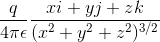whereis a constant.

Let S be a sphere in R3.

(a) Find the outward flux of E across S, if S does not contain q.

(b) Find the outward flux of E across S, if S is centered at q.

iyjzk 4TE (r2y 2)3/2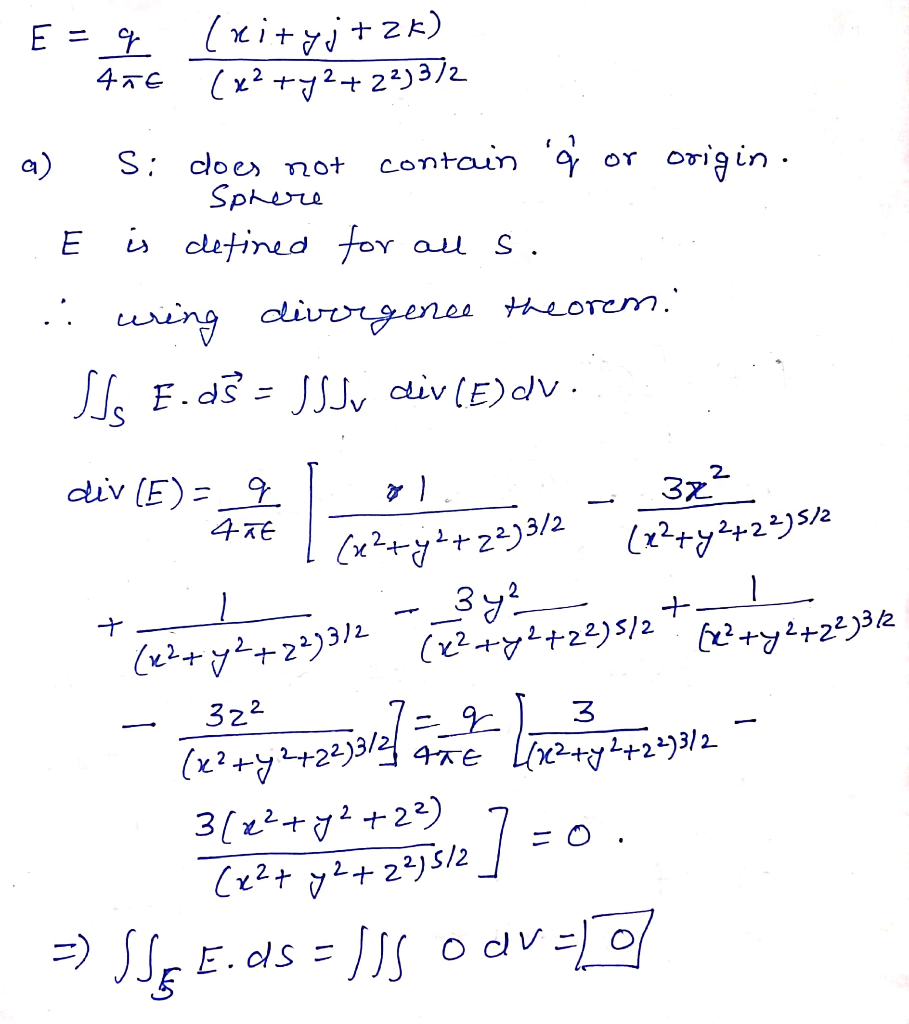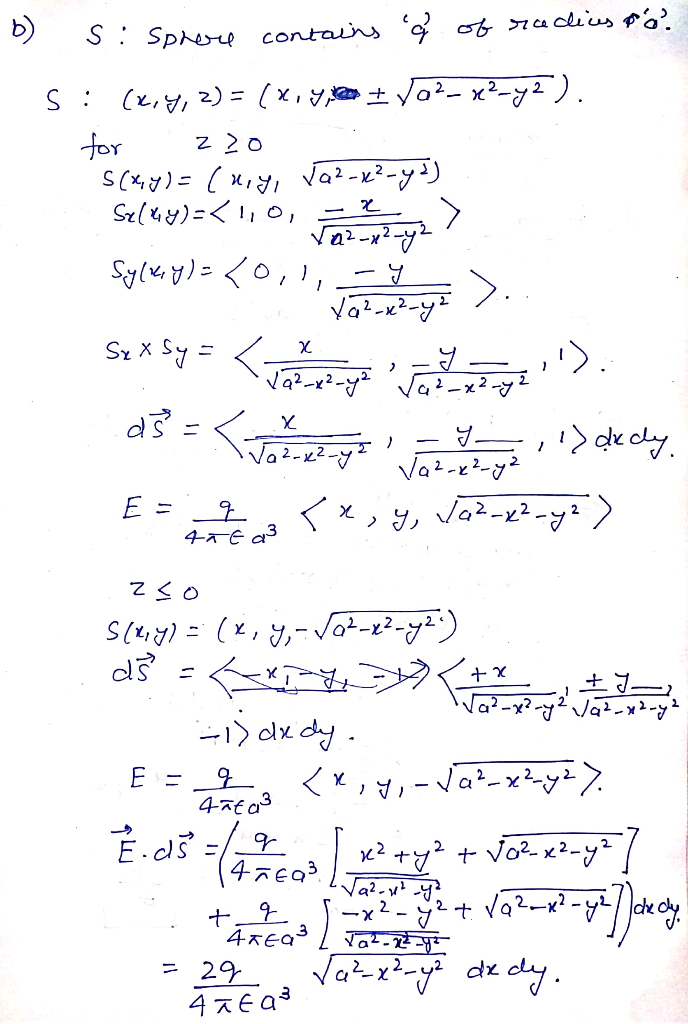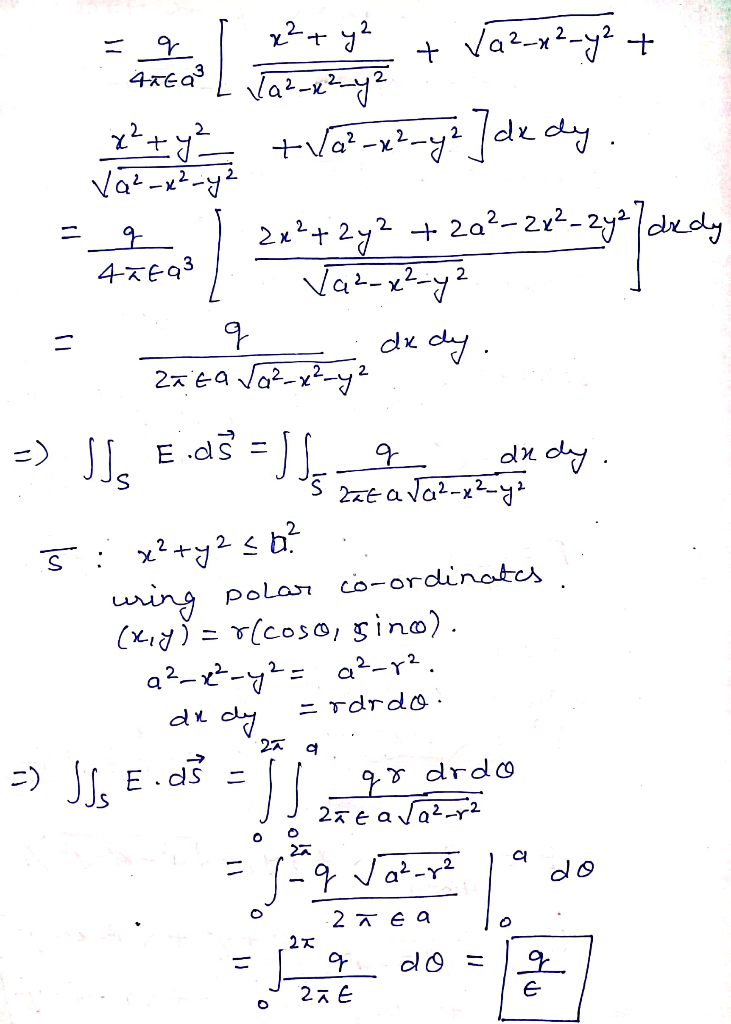##### Add Answer of: In electromagnetic theory, the electric eld created by a point charge q located at the origin is E = where is a const...
Similar Homework Help Questions
• ### 1) A point charge of 9.40 nC is located at the origin and a second charge...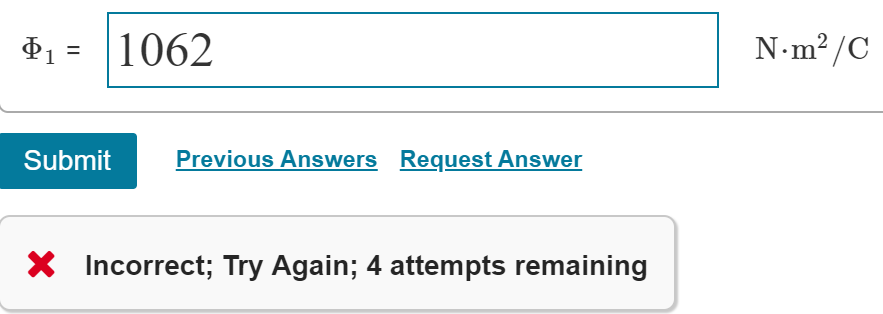1) A point charge of 9.40 nC is located at the origin and a second charge of -4.20 nC is located on the x axis at x=3.75cm. a) Calculate the electric flux through a sphere centered at the origin with radius 1.20 m . b) Repeat the calculation for a sphere of radius 2.70 m . 2) In a cubical volume, 0.70 m on a side, the electric field is   where E0=0.125N/C and a=0.70m. The cube has its sides parallel...

• ### 3) A Gaussian sphere of radius r is centered at the origin. A point charge q...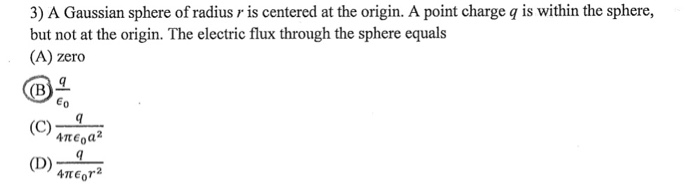3) A Gaussian sphere of radius r is centered at the origin. A point charge q is within the sphere, but not at the origin. The electric flux through the sphere equals (A) zero (O)méai (D) mCra

• ### Problem 5. (8 pts.) A point charge q, -5.00 nC is located at the origin, and...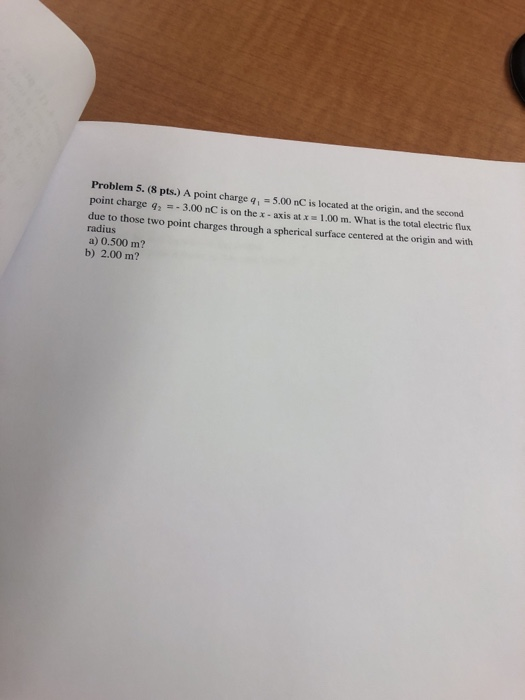Problem 5. (8 pts.) A point charge q, -5.00 nC is located at the origin, and the second point charge q3.00 nC is on the x -axis at x 1.00 m. What is the total electric flux due to thos radius a) 0.500 m? b) 2.00 m? e two point charges through a spherical surface centered at the origin and with

• ### A) Find electric field at origin (0,0) due to a negative charge q-2 nC located at position (0, 3m...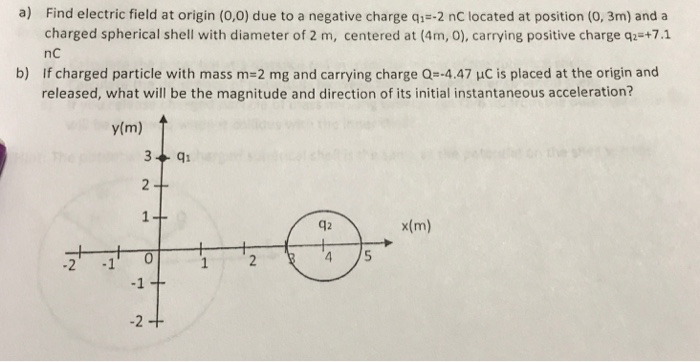a) Find electric field at origin (0,0) due to a negative charge q-2 nC located at position (0, 3m) and a nc charged spherical shell with diameter of 2 m, centered at (4m, 0), carrying positive charge q2 +7.1 If charged particle with mass m-2 mg and carrying charge Q=-4.47 μС ¡s placed at the origin and released, what will be the magnitude and direction of its initial instantaneous acceleration? b) y(m) 42 x(m) -2 1 -1 -2 a) Find...

• ### 3. a) Consider a point charge q at the center of an imaginary box with side...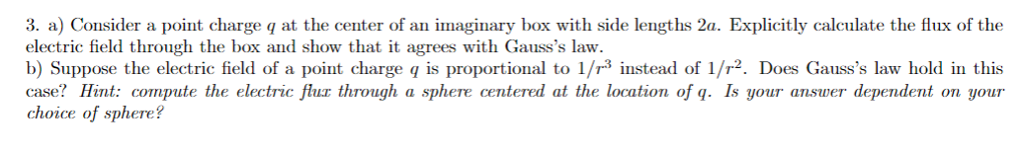3. a) Consider a point charge q at the center of an imaginary box with side lengths 2a. Explicitly calculate the flux of the electric field through the box and show that it agrees with Gauss's law. b) Suppose the electric field of a point charge q is proportional to 1/r3 instead of 1/2. Does Gauss's law hold in this case? Hint: compute the electric flur through a sphere centered at the location of q. Is your answer dependent on...

• ### A point charge Q [C] is located at a point in space. The charge is surrounded by two spherical la...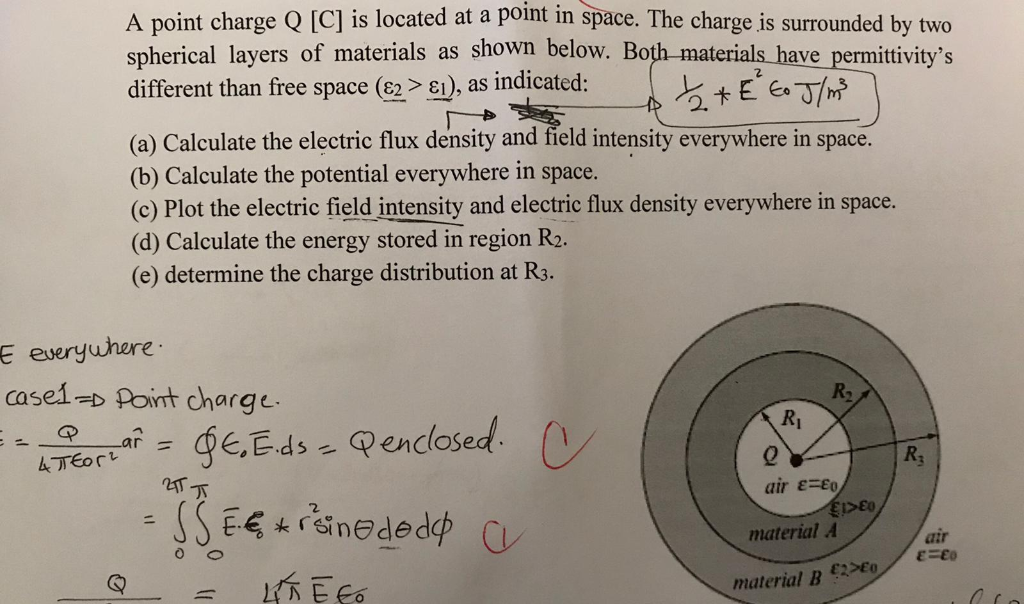please show steps and final answer A point charge Q [C] is located at a point in space. The charge is surrounded by two spherical layers of materials as shown below. Both-materials have permitivity's different than free space (82 > ε), as indicated: (a) Calculate the electric flux density and field intensity everywhere in space. (b) Calculate the potential everywhere in space. (c) Plot the electric field intensity and electric flux density everywhere in space. (d) Calculate the energy stored...

• ### A point charge q = +1 μC is located at the origin. What is the flux...

A point charge q = +1 μC is located at the origin. What is the flux of the electric field of this charge through a square whose corners are (x, y, z) = (1, 1, 1), (-1, 1, 1), (-1, 1, -1), and (1, 1, -1)? a. 0 b. 1.0 × 104 N m2/C c. 11.3 × 104 N m2/C d. 0.5 × 104 N m2/C e. 1.9 × 104 N m2/C

• ### A charge Q, located at the origin, produces an electric potential V = kQ/r at point...

A charge Q, located at the origin, produces an electric potential V = kQ/r at point r.  Which of the following statements concerning V is incorrect? Question 10 options: 1) The plane x = 5 is an equal-potential surface. 2) V is positive if Q is positive. 3) The spherical surface r = 5 is an equal-potential surface. 4) V is negative if Q is negative.

• ### electric field and flux 1.16 Gauss's law and two point charges* (a) Two point charges q...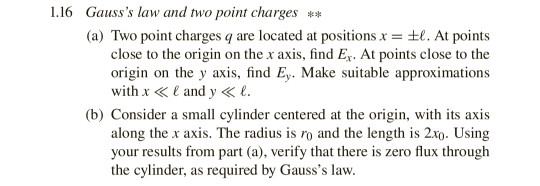electric field and flux 1.16 Gauss's law and two point charges* (a) Two point charges q are located at positions x = ±1. At points close to the origin on the x axis, find Ex. At points close to the origin on the y axis, find Ey. Make suitable approximations with x <E and y< e (b) Consider a small cylinder centered at the origin, with its axis along the x axis. The radius is ro and the length is...

• ### 2. A point charge q sits at the origin and a point charge 4q is located...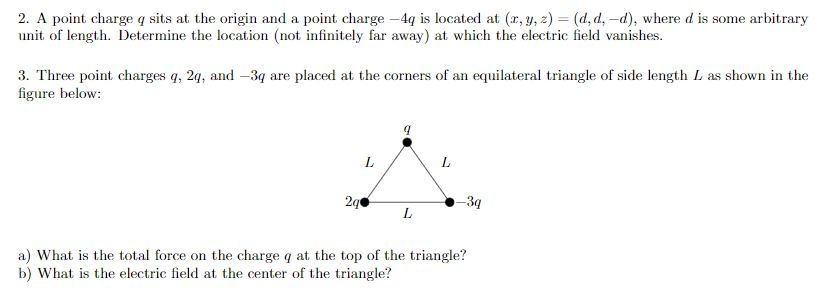2. A point charge q sits at the origin and a point charge 4q is located at (r, y, z) (d, d,-d), where d is some arbitrary unit of length. Determine the location (not infinitely far away) at which the electric field vanishes. 3. Three point charges q, 24, and -3q are placed at the corners of an equilateral triangle of side length L as shown in the figure below: 2q 3q a) What is the total force on the...

Need Online Homework Help?